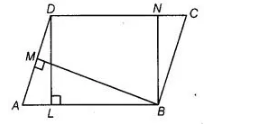# In the figure, the area of parallelogram $A B C D$ is`
Question:

In the figure, the area of parallelogram $A B C D$ is

(a) $A B \times B M$

(b) $\mathrm{BC} \times \mathrm{BN}$

(c) $D C \times D L$

(d) $A D \times D L$Thinking Process

Use the formula, area of parallelogram =B asex Altitude to get the required result

Solution:

(c) We know that, area of parallelogram is the product of its any side and the corresponding altitude (or height).

Here, when AB is base, then height is DL.

Area of parallelogram = AB x DL and when AD is base, then height is BM.

Area of parallelogram = AD x BM When DC is base, then height is DL.

Area of parallelogram = DC x DL and when BC is base, then height is not given.

Hence, option (c) is correct.